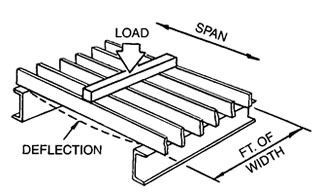This site requires javascript to be enabled to work properly.

Brown-Campbell Company
800-472-8464
Specialty Steel Manufacturer,
Fabricator & Distributor Since 1952
Product Information

## Bar Grating Load Tables

### Terminology & Formulas

Load Table Calculations are based on the following terminology and formulas:

#### Uniform Load#### Concentrated LoadU = Uniform Load - lbs/ft2

C = Concentrated Load - lbs/ft of grating width

D = Deflection - inches

F = Allowable Bending Stress
Light Duty: 18,000 psi
Heavy Duty: 20,000 psi
Aluminum: 12,000 psi
Stainless: 20,000 psi

E = Modulus of Elasticity, lbs. per sq. inch (psi)
Light Duty: 29,000,000 psi
Heavy Duty: 29,000,000 psi
Aluminum: 10,000,000 psi
Stainless: 28,000,000 psi

A = center to center distance between bearing bars, inches

K = number of bearing bars per foot of grating width = 12/A

t = Bearing Bar Thickness

d = Bearing Bar Depth

L = Simple Clear Span - feet

S = Section Modulus - in3/ft of grating width

I = Moment of Inertia - in4/bar

M = Bending Moment

a = Partial Load Contact Parallel to Span - inches

b = Partial Load Contact Dimension at 90 degrees to Span - inches; b = a + (2A)

P = Total Wheel or Partial Load Incl. Impact - lbs.

P1 = P per bearing bar; P1 = P x (A/b)

### Light Duty, Aluminum & Stainless Steel Load Calculation:

Uniform
Concentrated
Determine S:
S = Ktd2/6
S = Ktd2/6
Determine M:
M = FS/12
M = FS/12
Determine U or C:
U = 8M/L2
C = 4M/L
Determine l:
l = Ktd3/12
l = Ktd3/12
Check D*:
D = 5UL(Lx12)3
384EI
D = C(Lx12)3
48EI

* To ensure pedestrian comfort, maximum deflection should be limited to 1/4”.

### Heavy Duty Load Calculation:

Determine S:

S = Ktd2/6

Determine M:

M = FS/12

Substituting for M,
solve for L:

if a > L
then M = PL2/8ab

if a < L
then M = P(.25L-.125a)/b

Check D*:

D=P1[(2L3)-(a2L)+(a3/4)] / 96EI

* Maximum deflection should be limited to 1/400 span.